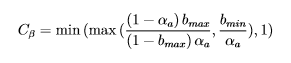# Python Calculator, finding maximum and minimum of two expressions to Output a field

Hello everyone,

I am trying to calculate a variable and show it as a point field in ParaView. I am using Python Calculator and until now, no luck. I am not sure what the problem is, since the code is correct.

The formula of the variable c_beta is as followswhere bmax and b min are 0.8 and 0.001 respectively
Here is the code I am using.

`````` minimum(maximum((1-PointData['alpha.air'])*0.8 / (1-0.8)*PointData['alpha.air']), 0.001/PointData['alpha.air']),1)
``````

Here is the state file:

cBeta.pvsm (1.0 MB)